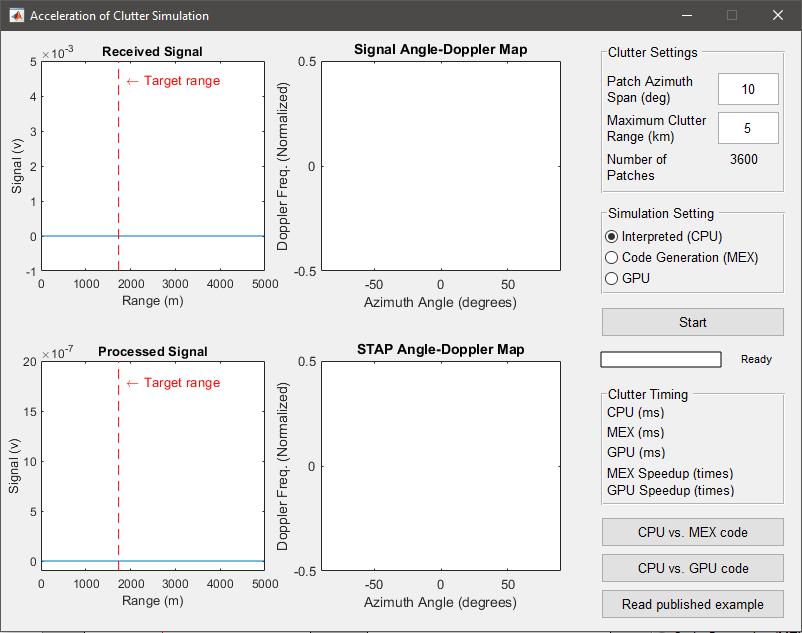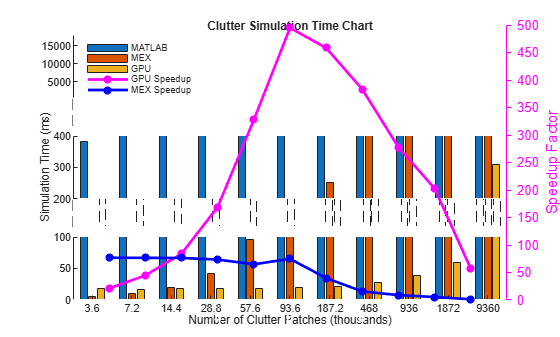Main Content

# Acceleration of Clutter Simulation Using GPU and Code Generation

This example shows how to simulate clutter on a graphical processing unit (GPU) or through code generation (MEX) instead of the MATLAB interpreter. The example applies the sample matrix inversion (SMI) algorithm, one of the popular space time adaptive processing (STAP) techniques, to the signal received by an airborne radar with a 6-element uniform linear array (ULA). The example focuses on comparing the performance of clutter simulation between GPU, code generation and the MATLAB interpreter. Interested readers can find details of the simulation and the algorithm in the example Introduction to Space-Time Adaptive Processing.

The full functionality of this example requires Parallel Computing Toolbox™ and MATLAB Coder™.

### Clutter Simulation

Radar system engineers often need to simulate the clutter return to test signal processing algorithms, such as STAP algorithms. However, generating a high fidelity clutter return involves many steps and therefore is often computationally expensive. For example, constantGammaClutter simulates the clutter using the following steps:

1. Divide the entire terrain into small clutter patches. The size of the patch depends on the azimuth patch span and the range resolution.

2. For each patch, calculate its corresponding parameters, such as the random return, the grazing angle, and the antenna array gain.

3. Combine returns from all clutter patches to generate the total clutter return.

The number of clutter patches depends on the terrain coverage, but it is usually in the range of thousands to millions. In addition, all steps above need to be performed for each pulse (assuming a pulsed radar is used). Therefore, clutter simulation is often the tall pole in a system simulation.

To improve the speed of the clutter simulation, one can take advantage of parallel computing. Note that the clutter return from later pulses could depend on the signal generated in earlier pulses, so certain parallel solutions offered by MATLAB, such as `parfor`, are not always applicable. However, because the computation at each patch is independent of the computations at other patches, it is suitable for GPU acceleration.

If you have a supported GPU and have access to Parallel Computing Toolbox, then you can take advantage of the GPU in generating the clutter return by using `gpuConstantGammaClutter` instead of c`onstantGammaClutter`. In most cases, using a `gpuConstantGammaClutter` System object is the only change you need to make.

If you have access to MATLAB Coder, you can also speed up clutter simulation by generating C code for c`onstantGammaClutter`, compiling it and running the compiled version. When running in code generation mode, this example compiles stapclutter using the codegen command:

``` codegen('stapclutter','-args',... {coder.Constant(maxRange),... coder.Constant(patchAzSpan)}); ```

All property values of c`onstantGammaClutter` have to be passed as constant values. The codegen command will generate the mex file, stapclutter_mex, which will be called in the loop.

### Comparing Clutter Simulation Times

To compare the clutter simulation performance between the MATLAB interpreter, code generation and a GPU, launch the following GUI by typing `stapcpugpu` in the MATLAB command line. The launched GUI is shown in the following figure:The left side of the GUI contains four plots, showing the raw received signal, the angle-Doppler response of the received signal, the processed signal, and the angle-Doppler response of the STAP processing weights. Again, the details can be found in the example Introduction to Space-Time Adaptive Processing. On the right side of the GUI, you control the number of clutter patches by modifying the clutter patch span in the azimuth direction (in degrees) and maximum clutter range (in km). You can then click the Start button to start the simulation, which simulates 5 coherent processing intervals (CPI) where each CPI contains 10 pulses. The processed signal and the angle-Doppler responses are updated once every CPI.

Next section shows timing for different simulation runs. In these simulations, each pulse consists of 200 range samples with a range resolution of 50 m. Combinations of the clutter patch span and the maximum clutter range result in various number of total clutter patches. For example, a clutter patch span of 10 degrees and a maximum clutter range of 5 km implies 3600 clutter patches. The simulations are carried out on the following system configurations:

• CPU: Xeon X5650, 2.66 GHz, 24 GB memory

• GPU: Tesla C2075, 6 GB memory

The timing results are shown in the following figure.

`helperCPUGPUResultPlot`From the figure, you can see that in general the GPU improves the simulation speed by dozens of times, sometimes even hundred of times. Two interesting observations are:

• When the number of clutter patches are small, as long as the data can be fit into the GPU memory, the GPU's performance is almost constant. The same is not true for the MATLAB interpreter.

• Once the number of clutter patches gets large, the data can no longer be fit into the GPU memory. Therefore, the speed up provided by GPU over the MATLAB interpreter starts to decrease. However, for close to ten millions of clutter patches, the GPU still provides an acceleration of over 50 times.

Simulation speed improvement due to code generation is less than the GPU speed improvement, but is still significant. Code generation for `constantGammaClutter` pre-calculates the collected clutter as an array of constant values. For larger number of clutter patches the size of the array becomes too big, thus reducing the speed improvement due to the overhead of memory management. Code generation requires access to MATLAB Coder but requires no special hardware.

### Other Simulation Timing Results

Even though the simulation used in this example calculates millions of clutter patches, the resulting data cube has a size of 200x6x10, indicating only 200 range samples within each pulse, 6 channels, and 10 pulses. This data cube is small compared to real problems. This example chooses these parameters to show the benefit you can get from using a GPU or code generation while ensuring that the example runs within a reasonable time in the MATLAB interpreter. Some simulations with larger data cube size yield the following results:

• 45-fold acceleration using a GPU for a simulation that generates 50 pulses for a 50-element ULA with 5000 range samples in each pulse, i.e., a 5000x50x50 data cube. The range resolution is 10 m. The radar covers a total azimuth of 60 degrees, with 1 degree in each clutter patch. The maximum clutter range is 50 km. The total number of clutter patches is 305,000.

• 60-fold acceleration using a GPU for a simulation like the one above, except with 180-degree azimuth coverage and a maximum clutter range equal to the horizon range (about 130 km). In this case, the total number of clutter patches is 2,356,801.

### Summary

This example compares the performance achieved by simulating clutter return using either the MATLAB interpreter, a GPU or code generation. The result indicates that the GPU and code generation offer big speed improvements over the MATLAB interpreter.

## SupportGet trial now